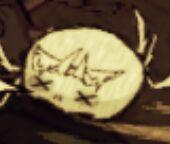# Why does the growtime of marble shrub in code differ from that of wiki explained?

## Recommended Posts

My friends, recently i started to read the code of some items and there is a question i met about the growtime of marble shrub.

In the file [marbleshrub.lua], there goes:

```local GROWTH_STAGES =
{
{
time = function(inst) return GetRandomWithVariance(TUNING.MARBLESHRUB_GROW_TIME.base, TUNING.MARBLESHRUB_GROW_TIME.random) end,
fn = function(inst) SetGrowth(inst) end,
growfn = function(inst) DoGrow(inst) end,
},
{
time = function(inst) return GetRandomWithVariance(TUNING.MARBLESHRUB_GROW_TIME.base, TUNING.MARBLESHRUB_GROW_TIME.random) end,
fn = function(inst) SetGrowth(inst) end,
growfn = function(inst) DoGrow(inst) end,
},
{
time = function(inst) return GetRandomWithVariance(TUNING.MARBLESHRUB_GROW_TIME.base, TUNING.MARBLESHRUB_GROW_TIME.random) end,
fn = function(inst) SetGrowth(inst) end,
growfn = function(inst) DoGrow(inst) end,
},
}```

the data of the 'time' in this code is:

```        MARBLESHRUB_GROW_TIME =
{
{base=9.0*day_time, random=1.0*day_time}, --short
{base=9.0*day_time, random=1.0*day_time}, --normal
{base=9.0*day_time, random=1.0*day_time}, --tall
},```

In the file [util.lua], function GetRandomWithVariance like below:

```function GetRandomWithVariance(baseval, randomval)
return baseval + (math.random()*2*randomval - randomval)
end```

From these codes I think the growtime of marble shrub should be 9±1 days. But I check it on wiki, it is far from what I think:

Quote

It takes 2.5-5 minutes for a Marble Sprout to grow to Stage 1, 5-6.25 days from Stage 1 to Stage 2, 5-6.25 days from Stage 2 to Stage 3, 5-6.25 days from Stage 3 to Stage 1. Growing is not affected by Seasons.

Why the growtime of marble shrub being 5-6.25 days? Is there more factor to effect this time that i have not noticed?

I'm confused about it now, anybody explain this to me? many thanks!!!!##### Share on other sites

From tuning.lua:

```local seg_time = 30
local day_segs = 10
local day_time = seg_time * day_segs```

The day_time variable is not the duration of an in-game day (which is 480 seconds). It is the standard length of the "day" portion of an in-game day AKA 300 seconds.

At each stage, the code calls GetRandomWithVariance(2700, 300), which returns

`2400 + math.random()*600`

Minimum value is 2400 =  5 days.

Maximum value is 3000 = 6.25 days.

##### Share on other sites

10 minutes ago, QuartzBeam said:

From tuning.lua:

```
local seg_time = 30
local day_segs = 10
local day_time = seg_time * day_segs```

The day_time variable is not the duration of an in-game day (which is 480 seconds). It is the standard length of the "day" portion of an in-game day AKA 300 seconds.

At each stage, the code calls GetRandomWithVariance(2700, 300), which returns

```
2400 + math.random()*600```

Minimum value is 2400 =  5 days.

Maximum value is 3000 = 6.25 days.

many thanks!!!! Thanks to ur explanation, I found the definition of the this data in [tuning.lua].

Quote

local seg_time = 30
local total_day_time = seg_time*16

local day_segs = 10
local dusk_segs = 4
local night_segs = 2

--default day composition. changes in winter, etc
local day_time = seg_time * day_segs
local dusk_time = seg_time * dusk_segs
local night_time = seg_time * night_segs

I used to think that the time of day,dusk and night should be a changeble data, so I never thought that 'day_time'  being a 10 seg_time data. It is a useful knowledge, thanks!